MCAT Physical : Electrochemistry

Example Questions

← Previous 1 3 4

Example Question #1 : Electrochemistry

In an electrolytic cell, oxidation takes place at the __________.

salt bridge

anode

cathode

Oxidation does not take place in an electrolytic cell.

anode

Explanation:

Electrolytic cells have a negative electromotive force and require an outside energy source to power a non-spontaneous reaction. Galvanic cells, in contrast, have positive potentials and facilitate spontaneous reactions without the need of a power source.

Regardless of cell type, however, oxidation always takes place at the anode and reduction always takes place at the cathode. The flow of electrons is always from the anode to cathode.

Example Question #1 : Electrochemistry

A student conducts an experiment for a chemistry class. The student wishes to explore power generation from different types of voltaic cells. He sets up three different cells, and then compares the amount of energy generated from each one.

One of his cells is shown below as an example. Both remaining cells follow the same layout.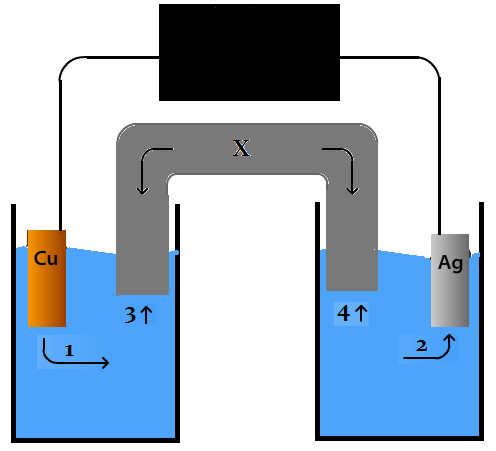How would the voltaic cell depicted above differ from an electrolytic cell?

An electrolytic cell requires energy input

An electrolytic cell produces more energy

An electrolytic cell produces more energy, but only after an input of energy to begin the process

An voltaic cell requires energy input to begin the reduction process

An electrolytic cell produces energy based on differences in concentrations between the half cells

An electrolytic cell requires energy input

Explanation:

Electrolytic cells require an input of energy, and are used to plate metals by functionally running a voltaic cell in reverse.

Example Question #3 : Electrolytic Cells

Which of the following is not true of electrolytic cells?

Electricity is conducted by the motion of ions

Oxidation occurs at the anode

Electrons travel toward the cathode

The cell potential is negative

No battery is required for electrolysis to take place

No battery is required for electrolysis to take place

Explanation:

For electrolytic cells, the cell potential is negative; in contrast, galvanic/voltaic cells have positive cell potentials. Electrolysis reactions can only occur if the total potential is positive. An additional voltage input, such as a battery, is required so that the sum of potentials in the electrolytic cell is greater than zero.

Oxidation always occurs at the anode, regardless of cell type, and electrons always travel toward the site of reduction (the cathode). In a galvanic cell, the cathode is positively charged, naturally drawing the flow of electrons. In an electrolytic cell, the cathode is negatively charged, but still requires the flow of electrons to allow reduction to occur. An induced current from a battery is used to propel these electrons against their natural flow.

Example Question #4 : Electrolytic Cells

Which of the following is true about electrolysis?

I. It only involves reactions with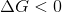II. It requires a voltage source

III. It is an exothermic process

II only

I only

II and III

I and II

II only

Explanation:

Electrolysis is a specific type of reaction that occurs in an electrolytic cell. An electrochemical cell contains an anode and a cathode that facilitate a redox reaction. In an electrolytic cell (a type of electrochemical cell) the redox reaction that is carried out is a nonspontaneous reaction. Recall that the change in Gibbs free energy for a nonspontaneous, or unfavorable, reaction is always positive; therefore, for electrolysis in an electrolytic cell, the redox reaction has a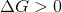. Statement I is false.

Nonspontaneous reactions are reactions that are unfavorable. This means that energy is required to carry out the reaction. In an electrolytic cell, energy is provided in the form of voltage input. The voltage provided pushes the reaction in the unfavorable direction; therefore, electrolysis reactions require a voltage source. Statement II is true.

Since it requires energy, an electrolysis reaction is considered to be an endothermic process. Recall that endothermic processes are reactions that take in (or require) energy, whereas exothermic processes are reactions that release energy; therefore, electrolysis is an endothermic process. Statement III is false.

Example Question #5 : Electrolytic Cells

Consider the following reaction: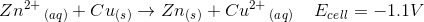How much voltage will you have to apply to carry out this reaction?

Less than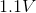No voltage needs to be applied because this reaction represents an electrolytic cell

Greater thanNo voltage needs to be applied because this reaction represents a galvanic cell

Greater thanExplanation:

The question states that the reaction has a negative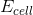; therefore, the reaction is nonspontaneous. Nonspontaneous reactions are carried out in electrolytic cells (as opposed to galvanic cells). A reaction usually proceeds in the spontaneous direction; therefore, to carry out nonspontaneous reactions you must put energy into the system. Without energy, the reaction shown will occur in the reverse direction.

In an electrolytic cell, energy is provided by an external voltage source. Without energy, the electrolytic cell will have a voltage ofand the spontaneous (reverse) reaction will occur. For the nonspontaneous reaction to occur, you must attach a voltage source in such a way that the voltage applied is greater thanand is applied in the opposite direction (nonspontaneous reaction direction). This will force the reaction in the reverse direction and the nonspontaneous reaction will occur; therefore, the external voltage source must provide a voltage greater than.

Example Question #1 : Electrochemistry

A student conducts an experiment for a chemistry class. The student wishes to explore power generation from different types of voltaic cells. He sets up three different cells, and then compares the amount of energy generated from each one.

One of his cells is shown below as an example. Both remaining cells follow the same layout.Instead of silver, a scientist uses a strip of zinc in the opposite half cell from copper. Which of the following is true when comparing this new cell to the cell in the diagram?

The reduction potential of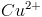is 0.34 volts. The reduction potential for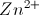is -0.76 volts.

The direction of electron flow is the same in both cells

Ions are no longer generated in the new cell

The cathode is the same in both cells

Energy can be produced in both cells

The direction of ion migration in the salt bridge is the same in both cells

Energy can be produced in both cells

Explanation:

In the new cell, energy can still be produced, but because zinc ions have a lower reduction potential than copper ions, the copper will be reduced and the direction of electron flow will be reversed, as compared to the cell with silver in which copper was oxidized.

Example Question #1 : Half Reactions And Reduction Potential

A student conducts an experiment for a chemistry class. The student wishes to explore power generation from different types of voltaic cells. He sets up three different cells, and then compares the amount of energy generated from each one.

One of his cells is shown below as an example. Both remaining cells follow the same layout.If there is a net production of copper ions in the half cell on the left as the reaction proceeds, which of the following must be true?

There is no net electron flow

Silver is oxidized in the reaction

Copper is reduced in the reaction

Copper has a more positive reduction potential than silver

Silver has a more positive reduction potential than copper

Silver has a more positive reduction potential than copper

Explanation:

If copper ions are generated as the voltaic cell functions, then the copper is being oxidized, and the silver must be reduced. Reduction and oxidation always occur together in a coupled reaction. This must also mean that the reduction potential for Ag is higher than the reduction potential for Cu.

Example Question #3 : Electrochemistry

A student conducts an experiment for a chemistry class. The student wishes to explore power generation from different types of voltaic cells. He sets up three different cells, and then compares the amount of energy generated from each one.

One of his cells is shown below as an example. Both remaining cells follow the same layout.As the difference in the reduction potentials between two half cells increases, what happens to the Gibbs free energy of the reaction?

It becomes more positive

It will increase or decrease, depending on the species involved

It does not change, as thermodynamics is independent of reduction potential

It only changes with changes in temperature or pressure, and is independent of the chemical species involved

It becomes more negative

It becomes more negative

Explanation:

The reduction potential of a cell is directly related to the Gibbs free energy by the equation below.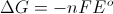As the reduction potential of a cell becomes more and more positive, the Gibbs free energy value becomes more and more negative.

Example Question #1 : Electrochemistry

Imagine a galvanic cell which uses solid zinc and aqueous iron ions to produce a voltage.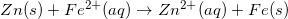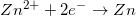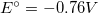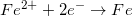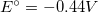What is the standard state cell potential for this reaction?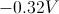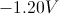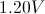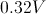Explanation:

Keep in mind that a galvanic cell will always have a positive voltage, so you can disregard the negative options. The half reactions show the voltage that will result if the element in question is reduced; however, an oxidation-reduction reaction will always have one element oxidized and another element reduced. In the equation shown, solid zinc (Zn) is oxidized, so the voltage of its half reaction is inverted to +0.76V. Next, you add the voltage of iron's reduction, resulting in the overall voltage of the galvanic cell.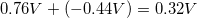Example Question #5 : Electrochemistry

Imagine a galvanic cell which uses solid zinc and aqueous iron ions to produce a voltage.Suppose that this galvanic cell was converted into an electrolytic cell. Which of the following statements would be true?

The cell potential would be negative

Oxidation would take place at the cathode

The reaction is spontaneous

No electrons would flow from anode to cathode